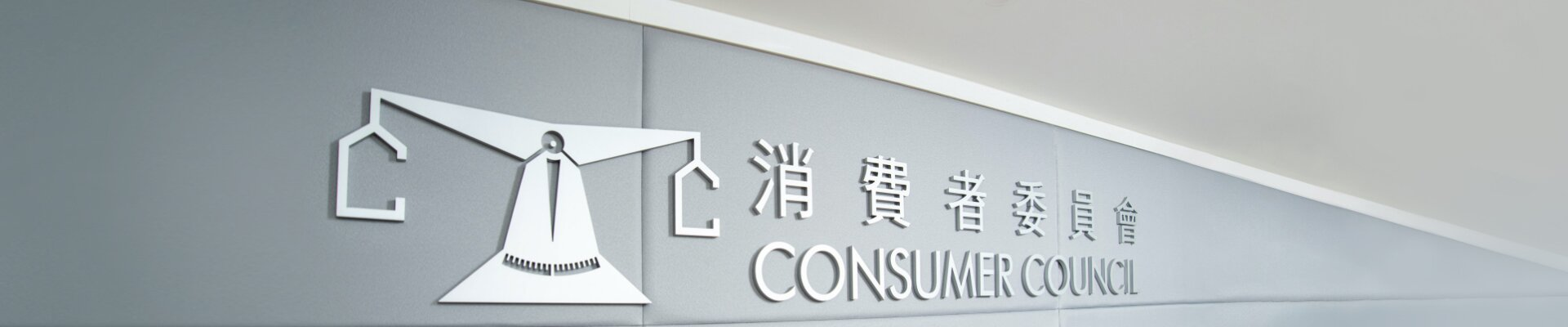• 陈建年先生
• 陈宝玲医生
• 周浩鼎议员
• 霍伟栋博士
• 冯洁鸣女士
• 何闻达先生
• 关静仪女士
•
• 林凯章先生，太平绅士
• 林建康律师，荣誉勋章，太平绅士
• 刘子芸女士
• 李泳兰女士
• 连少冬女士
• 雷绍麟先生
• 雷永昌医生
•
• 麦嘉晋先生
• 彭楚夫先生
• 萧景威先生
• 谭凤仪教授，铜紫荆星章，太平绅士
• 温丽司女士
• 余承章资深大律师
•
• 陈继宇博士，荣誉勋章，太平绅士
• 湛家扬博士
• 张国威副校长
• 张颕娴女士
• 车品觉教授，太平绅士
• 费中明律师，太平绅士
• 方奕展先生
• 郭晓晖先生
•
•
• 林绮薇女士
• 刘振鸿先生，荣誉勋章
• 刘恩沛大律师
• 刘业德先生
• 吴丽萍博士
• 潘释正教授
• 施永青先生，太平绅士
• 萧淑瑜大律师
• 谭巧莲博士
• 邓国伟先生
• 黄家伟博士
• 黄健威先生
• 黄蔚澄博士
• 黄宏辉先生
• 王永恺大律师
• 姚伟梅教授
•

• 陈锦荣先生，荣誉勋章，太平绅士
• 邝祖盛律师，荣誉勋章
• 何闻达先生
• 林建康律师，荣誉勋章，太平绅士
• 雷永昌医生
• 彭楚夫先生
• 谭凤仪教授，铜紫荆星章，太平绅士
•
• 邝祖盛律师，荣誉勋章
• 陈锦荣先生，荣誉勋章，太平绅士
• 冯洁鸣女士
• 何闻达先生
• 连少冬女士
• 张颕娴女士
•
•
•

• 余承章资深大律师
• 林建康律师，荣誉勋章，太平绅士
• 周浩鼎议员
• 关静仪女士
• 邝祖盛律师，荣誉勋章
• 林凯章先生，太平绅士
• 雷绍麟先生
•

•
• 费中明律师，太平绅士
• 刘恩沛大律师
• 施永青先生，太平绅士
• 萧淑瑜大律师
• 邓国伟先生
• 王永恺大律师
•

• 萧景威先生
• 李泳兰女士
• 陈建年先生
• 陈宝玲医生
• 冯洁鸣女士
• 林凯章先生，太平绅士
• 刘子芸女士
• 雷绍麟先生
• 麦嘉晋先生
• 彭楚夫先生
• 温丽司女士
•
• 陈继宇博士，荣誉勋章，太平绅士
•
• 谭凤仪教授，铜紫荆星章，太平绅士
• 雷永昌医生
• 陈宝玲医生
• 霍伟栋博士
• 刘子芸女士
• 李泳兰女士
• 连少冬女士
• 麦嘉晋先生
• 萧景威先生
• 温丽司女士
•
• 邝祖盛律师，荣誉勋章
• 彭楚夫先生
• 陈建年先生
• 周浩鼎议员
• 冯洁鸣女士
• 关静仪女士
• 林凯章先生，太平绅士
• 雷绍麟先生
• 余承章资深大律师
•
• 郭晓晖先生
• 吴丽萍博士
• 萧景威先生
• 麦嘉晋先生
• 陈宝玲医生
• 霍伟栋博士
• 冯洁鸣女士
• 刘子芸女士
• 连少冬女士
•
• 陈继宇博士，荣誉勋章，太平绅士
• 湛家扬博士
• 车品觉教授，太平绅士
• 潘释正教授
•
• 谭凤仪教授，铜紫荆星章，太平绅士
• 陈建年先生
• 何闻达先生
• 关静仪女士
• 刘子芸女士
• 李泳兰女士
• 萧景威先生
• 温丽司女士
• 余承章资深大律师
• 吴丽萍博士
•

• 彭楚夫先生

• 张国威副校长
• 方奕展先生
• 林绮薇女士
• 刘振鸿先生，荣誉勋章
• 刘业德先生
• 谭巧莲博士
• 黄家伟博士
• 黄健威先生
• 黄蔚澄博士
• 黄宏辉先生
• 姚伟梅教授